Prealgebra 2e

# Review Exercises

Prealgebra 2eReview Exercises

### Review Exercises

##### Use the Rectangular Coordinate System

Plot Points in a Rectangular Coordinate System

In the following exercises, plot each point in a rectangular coordinate system.

277.

$( 1 , 3 ) , ( 3 , 1 ) ( 1 , 3 ) , ( 3 , 1 )$

278.

$( 2 , 5 ) , ( 5 , 2 ) ( 2 , 5 ) , ( 5 , 2 )$

In the following exercises, plot each point in a rectangular coordinate system and identify the quadrant in which the point is located.

279.
1. $(−1,−5)(−1,−5)$
2. $(−3,4)(−3,4)$
3. $(2,−3)(2,−3)$
4. $(1,52)(1,52)$
280.
1. $(3,−2)(3,−2)$
2. $(−4,−1)(−4,−1)$
3. $(−5,4)(−5,4)$
4. $(2,103)(2,103)$

Identify Points on a Graph

In the following exercises, name the ordered pair of each point shown in the rectangular coordinate system.

281.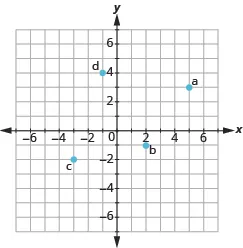282.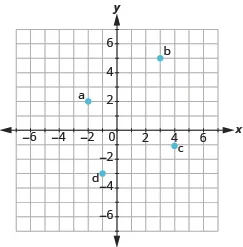283.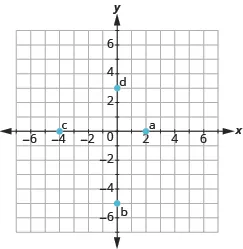284.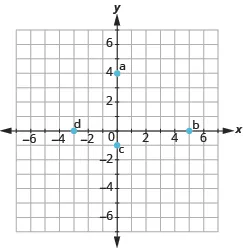Verify Solutions to an Equation in Two Variables

In the following exercises, find the ordered pairs that are solutions to the given equation.

285.

$5 x + y = 10 5 x + y = 10$

1. $(5,1)(5,1)$
2. $(2,0)(2,0)$
3. $(4,−10)(4,−10)$
286.

$y = 6 x − 2 y = 6 x − 2$

1. $(1,4)(1,4)$
2. $(13,0)(13,0)$
3. $(6,−2)(6,−2)$

Complete a Table of Solutions to a Linear Equation in Two Variables

In the following exercises, complete the table to find solutions to each linear equation.

287.

$y = 4 x − 1 y = 4 x − 1$

$xx$ $yy$ $(x,y)(x,y)$
$00$
$11$
$−2−2$
288.

$y = − 1 2 x + 3 y = − 1 2 x + 3$

$xx$ $yy$ $(x,y)(x,y)$
$00$
$11$
$−2−2$
289.

$x + 2 y = 5 x + 2 y = 5$

$xx$ $yy$ $(x,y)(x,y)$
$00$
$11$
$−1−1$
290.

$3 x − 2 y = 6 3 x − 2 y = 6$

$xx$ $yy$ $(x,y)(x,y)$
$00$
$00$
$−2−2$

Find Solutions to a Linear Equation in Two Variables

In the following exercises, find three solutions to each linear equation.

291.

$x + y = 3 x + y = 3$

292.

$x + y = −4 x + y = −4$

293.

$y = 3 x + 1 y = 3 x + 1$

294.

$y = − x − 1 y = − x − 1$

##### Graphing Linear Equations

Recognize the Relation Between the Solutions of an Equation and its Graph

In each of the following exercises, an equation and its graph is shown. For each ordered pair, decide

1. if the ordered pair is a solution to the equation.
2. if the point is on the line.
295.

$y=−x+4y=−x+4$1. $(0,4)(0,4)$
2. $(−1,3)(−1,3)$
3. $(2,2)(2,2)$
4. $(−2,6)(−2,6)$
296.

$y=23x−1y=23x−1$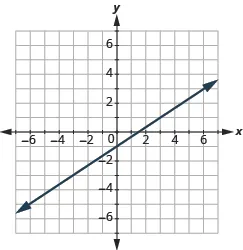1. $(0,−1)(0,−1)$
2. $(3,1)(3,1)$
3. $(−3,−3)(−3,−3)$
4. $(6,4)(6,4)$

Graph a Linear Equation by Plotting Points

In the following exercises, graph by plotting points.

297.

$y = 4 x − 3 y = 4 x − 3$

298.

$y = −3 x y = −3 x$

299.

$2 x + y = 7 2 x + y = 7$

Graph Vertical and Horizontal lines

In the following exercises, graph the vertical or horizontal lines.

300.

$y = −2 y = −2$

301.

$x = 3 x = 3$

##### Graphing with Intercepts

Identify the Intercepts on a Graph

In the following exercises, find the $x-x-$ and $y-intercepts.y-intercepts.$

302.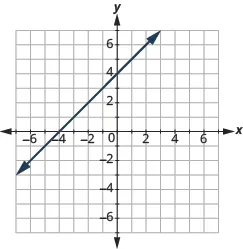303.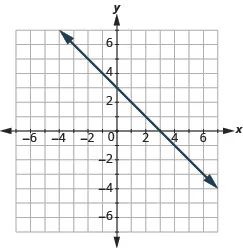Find the Intercepts from an Equation of a Line

In the following exercises, find the intercepts.

304.

$x + y = 5 x + y = 5$

305.

$x − y = −1 x − y = −1$

306.

$y = 3 4 x − 12 y = 3 4 x − 12$

307.

$y = 3 x y = 3 x$

Graph a Line Using the Intercepts

In the following exercises, graph using the intercepts.

308.

$− x + 3 y = 3 − x + 3 y = 3$

309.

$x + y = −2 x + y = −2$

Choose the Most Convenient Method to Graph a Line

In the following exercises, identify the most convenient method to graph each line.

310.

$x = 5 x = 5$

311.

$y = −3 y = −3$

312.

$2 x + y = 5 2 x + y = 5$

313.

$x − y = 2 x − y = 2$

314.

$y = 1 2 x + 2 y = 1 2 x + 2$

315.

$y = 3 4 x − 1 y = 3 4 x − 1$

##### Understand Slope of a Line

Use Geoboards to Model Slope

In the following exercises, find the slope modeled on each geoboard.

316.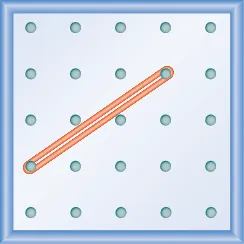317.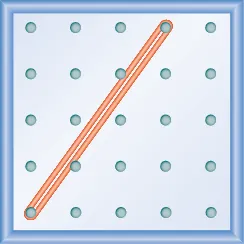318.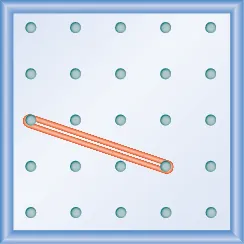319.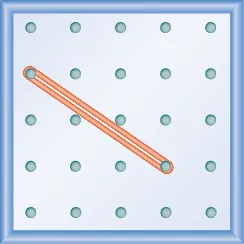In the following exercises, model each slope. Draw a picture to show your results.

320.

$1 3 1 3$

321.

$3 2 3 2$

322.

$− 2 3 − 2 3$

323.

$− 1 2 − 1 2$

Find the Slope of a Line from its Graph

In the following exercises, find the slope of each line shown.

324.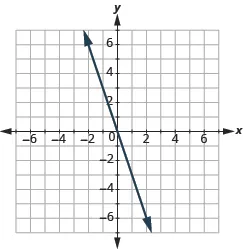325.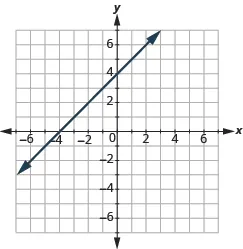326.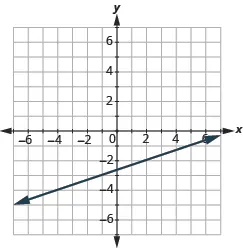327.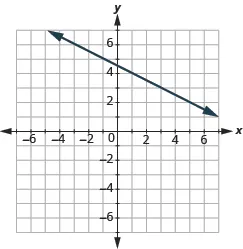Find the Slope of Horizontal and Vertical Lines

In the following exercises, find the slope of each line.

328.

$y = 2 y = 2$

329.

$x = 5 x = 5$

330.

$x = −3 x = −3$

331.

$y = −1 y = −1$

Use the Slope Formula to find the Slope of a Line between Two Points

In the following exercises, use the slope formula to find the slope of the line between each pair of points.

332.

$( 2 , 1 ) , ( 4 , 5 ) ( 2 , 1 ) , ( 4 , 5 )$

333.

$( −1 , −1 ) , ( 0 , −5 ) ( −1 , −1 ) , ( 0 , −5 )$

334.

$( 3 , 5 ) , ( 4 , −1 ) ( 3 , 5 ) , ( 4 , −1 )$

335.

$( −5 , −2 ) , ( 3 , 2 ) ( −5 , −2 ) , ( 3 , 2 )$

Graph a Line Given a Point and the Slope

In the following exercises, graph the line given a point and the slope.

336.

$( 2 , −2 ) ; m = 5 2 ( 2 , −2 ) ; m = 5 2$

337.

$( −3 , 4 ) ; m = − 1 3 ( −3 , 4 ) ; m = − 1 3$

Solve Slope Applications

In the following exercise, solve the slope application.

338.

A roof has rise $1010$ feet and run $1515$ feet. What is its slope?

Order a print copy

As an Amazon Associate we earn from qualifying purchases.